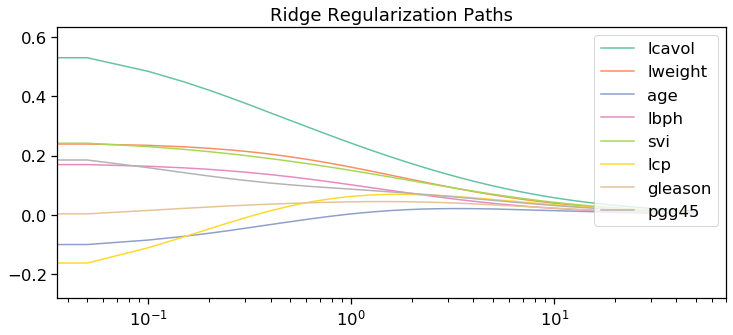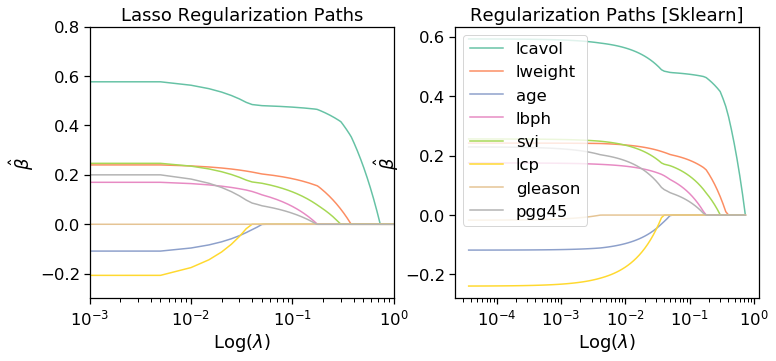# Shrinkage methods: Ridge and LASSO

## Ridge Regression

Ridge regression shrinks coefficients, $$\hat{\beta}$$, by penalizing the residual sum of squares with a Lagrange multiplier, $$\lambda$$.

$\hat{\beta}^{ridge} = \operatorname*{arg\,min}_\beta \bigg\{ \sum_{i=1}^{N}\big(y_i - \beta_0 - \sum_{j=1}^{p} x_{ij}\beta_j\big)^2 +\lambda \sum_{j=1}^{p} \beta^2_j\bigg\}$

Written as the optimization,

$\hat{\beta}^{ridge} = \operatorname*{arg\,min}_\beta \sum_{i=1}^{N}\big(y_i - \beta_0 - \sum_{j=1}^{p} x_{ij}\beta_j\big)^2,\\ \sum_{j=1}^{p} \beta^2_j\leq t$

for some $$t>0$$. In matrix notation, the Lagrangian is written as,

$L(X,t,\lambda^*,\beta ) =\|Y - X\beta\|_2^2 + \lambda^*(\|\beta\|_2^2 - t)$

where $$\|\bullet\|$$ denotes the $$L_2$$ norm. The optimal solution for the estimator, $$\hat{\beta}^{ridge}$$, is proved with Karush-Kuhn-Tucker (KKT) conditions, $$\nabla_{\beta} L(X,c,\lambda^*,\beta ) = 0$$ (stationarity) and $$\lambda^*(\|\beta(\lambda^*)\|_2^2 - t) = 0$$ (Complementary slackness ). With the KKT conditions,

$-2X^T(Y-X\beta) + 2t\beta = 0,\\ \lambda^*(\|\beta(\lambda^*)\|_2^2 - t) = 0$

Both conditions are satisfied if $$\lambda^* = \lambda$$ and $$t = \lambda^*\|\beta(\lambda^*)\|_2^2$$.

The solution to the ridge estimator is solved similar to linear regression.

$RSS = (Y-\beta X)^T(Y-\beta X) - \lambda \beta^T \beta$

where the solution is

$\hat{\beta}^{ridge} = (X^TX - \lambda I)^{-1} X^T Y$

## LASSO Regression

Lasso regression is similar to ridge regression however $$L_2$$ penalty is replaced by the $$L_1$$ penalty.

$\hat{\beta}^{ridge} = \operatorname*{arg\,min}_\beta \sum_{i=1}^{N}\big(y_i - \beta_0 - \sum_{j=1}^{p} x_{ij}\beta_j\big)^2,\\ \sum_{j=1}^{p} \mid\beta_j\mid \leq t$

Coordinated Decent is an efficient method for solving ridge and LASSO problems.

### Coordinated Descent (Algorithim):

Coordinate descent is done by iteratively regressing a predictor, $$X_j$$, on all currently explained predictors $$X_{-j}$$. $$X\beta$$ can be split into $$Y = X_j\beta_j + X_{-j}\beta_{-j}$$.

$Y = X_j\beta_j + X_{-j}\beta_{-j}\\ X_j \beta_j = y - x_{-j}\beta_{-j}\\ X_j^T X_j \beta_j = X_j^T (y - x_{-j}\beta_{-j})\\ \beta_j = \frac{X_j^T}{X_j^T X_j }(y - x_{-j}\beta_{-j})$

One cycle of coordinate descent iterates over each predictor. For a given set of parameters this is repeated. Typically, the algorithim is run for a value of $$\lambda$$ such that $$\beta(\lambda_{max}) = 0$$. This is repeated for a smaller value of $$\lambda$$ using the previous of $$\lambda$$ as a ‘warm-start’.

%matplotlib inline
import numpy as np
import matplotlib.pyplot as plt
from sklearn import datasets
import pandas as pd
import seaborn as sns
sns.set_context("notebook", font_scale=1.5, rc={"lines.linewidth": 1.5})

#for comparision
from sklearn import linear_model
from sklearn import datasets

import warnings
warnings.filterwarnings("ignore")

#testing with the prostate dataset

#split train and test
df_train = df.loc[df['train'] == 'T']
df_test = df.loc[df['train'] == 'F']
#drop train column
df_train = df_train.drop(['train'],axis=1)
df_test = df_test.drop(['train'],axis=1)
x_train = df_train[['lcavol', 'lweight', 'age', 'lbph', 'svi', 'lcp', 'gleason', 'pgg45']].to_numpy()
y_train = df_train[['lpsa']].to_numpy()
x_test = df_test[['lcavol', 'lweight', 'age', 'lbph', 'svi', 'lcp', 'gleason', 'pgg45']].to_numpy()
y_test = df_test[['lpsa']].to_numpy()
predictors = ['lcavol', 'lweight', 'age', 'lbph', 'svi', 'lcp', 'gleason', 'pgg45']
p = len(predictors)
cs = sns.color_palette("Set2", p)

#for ridge regression standardize the predictors
x_train = (x_train - np.mean(x_train,axis=0))/np.std(x_train,axis=0)
x_test = (x_test - np.mean(x_test,axis=0))/np.std(x_test,axis=0)

y_train = (y_train - np.mean(y_train,axis=0))/np.std(y_train,axis=0)
y_train = (y_train - np.mean(y_train,axis=0))/np.std(y_train,axis=0)

#Method Use Coordinate descent to solve for betas
import copy
beta = np.zeros((x_train.shape,1))

#Lasso coefficient/L1
def soft_threshold_l1(t : float,_lambda : float) -> float:
m = abs(t)
if t > 0 and m > _lambda:
return m - _lambda
elif t < 0 and m > _lambda:
return _lambda - m
else:
return 0.0

#Ridge coefficient/L2
def soft_threshold_l2(t : float ,_lambda : float) -> float:
return t / (1.0+_lambda)

#Coordinate descent
def coordinate_descent(lambda_range,x_train, y_train, beta, iterations=100, model = 'lasso'):
#Iterative Over Lambdas
#then decrease to warm start the solution
for k,lval in enumerate(lambda_range):
#initalize r values
r = y_train - x_train @ beta
for i in range(iterations):

#iterate over predictors
for j in range(beta.shape):
temp_beta = copy.deepcopy(beta)
temp_beta[j] = 0.0
r = y_train - x_train @ temp_beta
xtx = np.sum(x_train[:,j]**2)
betaj = (1/xtx) * (x_train[:,j].T @ r)
if model == 'lasso':
beta[j] = soft_threshold_l1(betaj,lval)
elif model == 'ridge':
beta[j] = soft_threshold_l2(betaj,lval)

lambda_sol[k] = beta.T
return lambda_sol


#Ridge Solutions
lambda_tot = 1000
lambda_sol = np.zeros((lambda_tot,beta.shape))
lambda_range = np.arange(0,50,50.0/lambda_tot)[::-1]
lambda_sol = coordinate_descent(lambda_range, x_train, y_train, beta, model = 'ridge')

m,n = x_train.shape

#Solution via coordinate_descent method
fig, ax = plt.subplots(1,1,figsize=(12,5))
x = lambda_range
ax.set_title("L2/Ridge Regularization Path")
for i in range(n):
ax.semilogx(x, lambda_sol[:,i],color=cs[i],label=predictors[i])

ax.set_title('Ridge Regularization Paths')
ax.legend(loc='upper right')
plt.axis('tight')

(0.035399060013482114,
70.55272086458793,
-0.28090154214315,
0.6347657236127785)#Lasso Solutions
lambda_tot = 200
lambda_sol = np.zeros((lambda_tot,beta.shape))
lambda_range = np.arange(0,1,1.0/lambda_tot)[::-1]
lambda_sol = coordinate_descent(lambda_range, x_train, y_train, beta, model = 'lasso')

m,n = x_train.shape

#Solution via coordinate_descent method
fig, ax = plt.subplots(1,2,figsize=(12,5))
x = lambda_range
ax.set_title("L1/LASSO Regularization Path")
for i in range(n):
ax.semilogx(x, lambda_sol[:,i],color=cs[i],label=predictors[i])

#sklearn validation
alphas_lasso, coefs_lasso, _ = linear_model.lasso_path(x_train, y_train,\
5e-5 , fit_intercept=False)
for i in range(n):
ax.semilogx(alphas_lasso, coefs_lasso[i],\
label = predictors[i], color=cs[i])

for a in ax:
a.set_xlim((0.001,1))
a.set_ylim((-0.3,0.8))
a.set_xlabel(r"Log($\lambda$)")
a.set_ylabel(r"$\hat{\beta}$")

ax.set_title('Lasso Regularization Paths')
ax.set_title('Regularization Paths [Sklearn]')
ax.legend()
plt.axis('tight')

(2.2341606180573485e-05,
1.2029494762168695,
-0.28064122726898855,
0.6346202023890164)Updated: